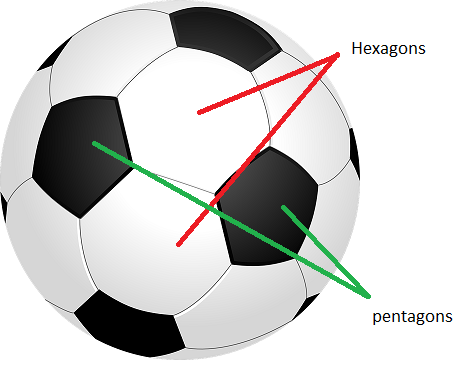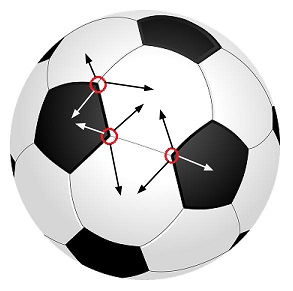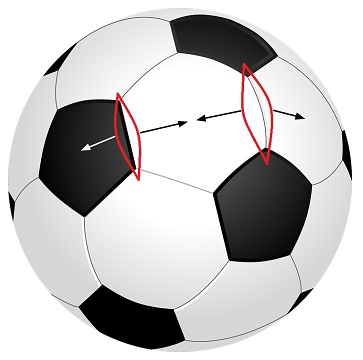# Number of Pentagons and Hexagons on a Football

Given a standard football, regular hexagons and pentagons are drawn on it as shown in the picture. Find out the number of hexagons and pentagons.## Recommended: Please try your approach on {IDE} first, before moving on to the solution.

We can apply Euler Characteristics to find out number of Hexagons and Pentagons on a standard Football.
According to Euler Characteristics: For every surface S there exists an integersuch that whenever a graph G with V vertices and E edges is embedded in S so that there are F faces(regions divided by the graph), we have:For a sphere(shape of football)= 2.
Hence, the equation becomes V – E + F = 2.

Now, let number of pentagons P and number of hexagons H.

Number of vertices will be:
6 vertices for each of the hexagons, i.e. 6*H.
5 vertices for each of the pentagons, i.e. 5*P.
But we have counted each vertex thrice, once for each adjacent polygon, follow the pictureHence, number of vertices, V = (6*H + 5*P)/3.

Number of edges will be:
6 edges for each of the hexagons, i.e. 6*H.
5 edges for each of the pentagons, i.e. 5*P.
But we have counted each edge twice, once for each adjacent polygon, follow the pictureHence, number of edges, E = (6*H + 5*P)/2.

Number of faces will be:
There are H hexagons and P pentagons, each forming a face. Hence, total number of faces, F = (H + P).

So, we can write:after solving this equation we will get P = 12. So, there are 12 Pentagons.
Now number of Hexagons:
We can see that each pentagon is surrounded by 5 Hexagons. So there should 5*P hexagons, but we have counted each hexagon thrice for each of its 3 adjacent pentagons. Hence, number of hexagons = 5*P/3 = 5*12/3 = 20.

Hence, there are 20 Hexagons and 12 Pentagons in a standard football.

Attention reader! Don’t stop learning now. Get hold of all the important DSA concepts with the DSA Self Paced Course at a student-friendly price and become industry ready.

My Personal Notes arrow_drop_upI am an undergrad at IIEST Shibpur love to code and solve algorithm data structure problems

If you like GeeksforGeeks and would like to contribute, you can also write an article using contribute.geeksforgeeks.org or mail your article to contribute@geeksforgeeks.org. See your article appearing on the GeeksforGeeks main page and help other Geeks.

Please Improve this article if you find anything incorrect by clicking on the "Improve Article" button below.